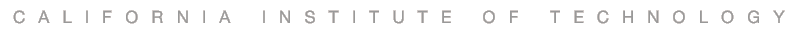# L E C T U R E S

The following lectures on machine learning were given by Professor Abu-Mostafa in his Learning From Data telecourse. You can also look for a particular topic in the Machine Learning Video Library.

• The Learning Problem - Introduction; supervised, unsupervised, and reinforcement learning. Components of the learning problem.

• Is Learning Feasible? - Can we generalize from a limited sample to the entire space? Relationship between in-sample and out-of-sample.

• The Linear Model I - Linear classification and linear regression. Extending linear models through nonlinear transforms.

• Error and Noise - The principled choice of error measures. What happens when the target we want to learn is noisy.

• Training versus Testing - The difference between training and testing in mathematical terms. What makes a learning model able to generalize?

• Theory of Generalization - How an infinite model can learn from a finite sample. The most important theoretical result in machine learning.

• The VC Dimension - A measure of what it takes a model to learn. Relationship to the number of parameters and degrees of freedom.

• Bias-Variance Tradeoff - Breaking down the learning performance into competing quantities. The learning curves.

• The Linear Model II - More about linear models. Logistic regression, maximum likelihood, and gradient descent.

• Neural Networks - A biologically inspired model. The efficient backpropagation learning algorithm. Hidden layers.

• Overfitting - Fitting the data too well; fitting the noise. Deterministic noise versus stochastic noise.

• Regularization - Putting the brakes on fitting the noise. Hard and soft constraints. Augmented error and weight decay.

• Validation - Taking a peek out of sample. Model selection and data contamination. Cross validation.

• Support Vector Machines - One of the most successful learning algorithms; getting a complex model at the price of a simple one.

• Kernel Methods - Extending SVM to infinite-dimensional spaces using the kernel trick, and to non-separable data using soft margins.

• Radial Basis Functions - An important learning model that connects several machine learning models and techniques.

• Three Learning Principles - Major pitfalls for machine learning practitioners; Occam's razor, sampling bias, and data snooping.

• Epilogue - The map of machine learning. Brief views of Bayesian learning and aggregation methods.